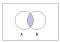# A couple proofs of Bayes Rule

Please don’t click away if you prefer “Bayes Law” or “Bayes Formula”.

The point of this post is to use the foundations of probability theory to prove the foundation of Bayesian statistics — Bayes Rule.

Here is a list of basic properties we will need. We are assuming A and B are two events.

Bayes Rule can be derived from this following relation, which will be explored in the next post:

The above relation reads as “Probability of Event A occurring , given Event B occurred equals the Probability of Event B occurring, given A occurred”.

At this point, I had two questions. 1) How did the result above come to be? and 2) Does it matter if A and B are (in)dependent?

2) can be answered by the following proofs:

2a) Assume A and B are dependent

2b) Assume A and B are independent

The answer to 2) is no.

This is due to the Commutative Intersection Property listed above, which answers 1). Both proofs use this property as a hinge (you can check!).If you switch the position of A and B, is their intersection affected? (Image Credit: https://www.thoughtco.com/intersection-in-set-theory-3126587)

These are just a couple proofs. It is possible to prove Bayes Rule without using Joint Probability as well.

EDIT: 2/14/21

I never actually mention Bayes Rule explicitly anywhere in the post, so here is the straightforward proof after having the knowledge and satisfaction of proving P(A,B) = P(B,A):